Refer to our Texas Go Math Grade 2 Answer Key Pdf to score good marks in the exams. Test yourself by practicing the problems from Texas Go Math Grade 2 Module 15 Assessment Answer Key.

Concepts and Skills

Circle the objects that match the name of the solid. TEKS 2.8.B

Question 1.
cylinder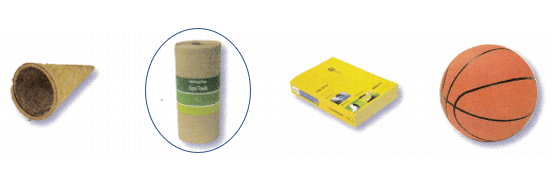Question 2.
cube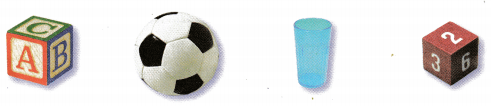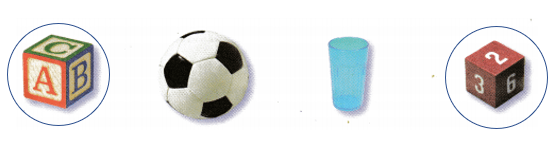Question 3.
cone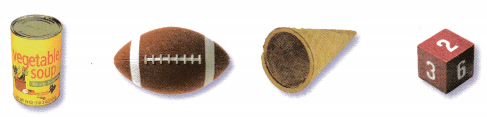Build a rectangular prism with the given number of unit cubes. Shade to show the top and front views. TEKS 2.8.D

Question 4.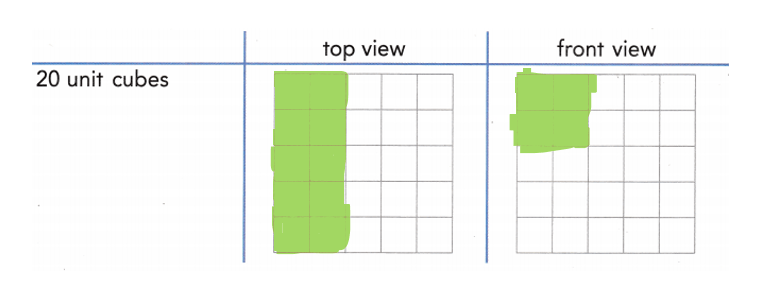Texas Test Prep

Fill in the bubble for the correct answer choice.

Question 5.
Which solid has 5 faces, 9 edges, and 6 vertices? TEKS 2.8.B.
(A) rectangular prism
(B) triangular prism
(C) cylinder
B. Triangular prism

Question 6.
Which name matches the solid shown? TEKS 2.8.B(A) cube
(B) cone
(C) cylinder
B. Cone

Question 7.
Nora built a rectangular prism using unit cubes. How many unit cubs did Nora use? TEKS 2.8.D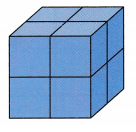(A) 12
(B) 8
(C) 6
B. 8

Question 8.
Kevin has a cube. How many vertices does a cube have? TEKS 2.8.B
(A) 6
(B) 16
(C) 8